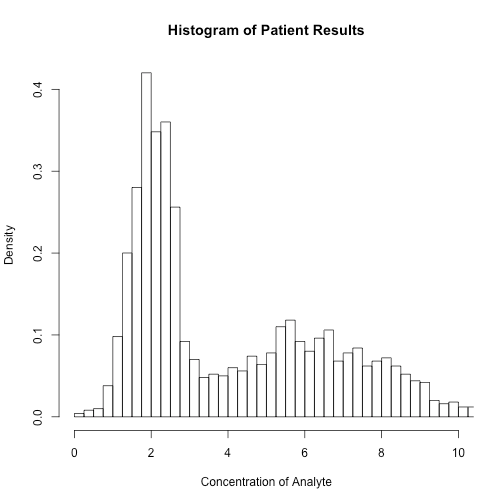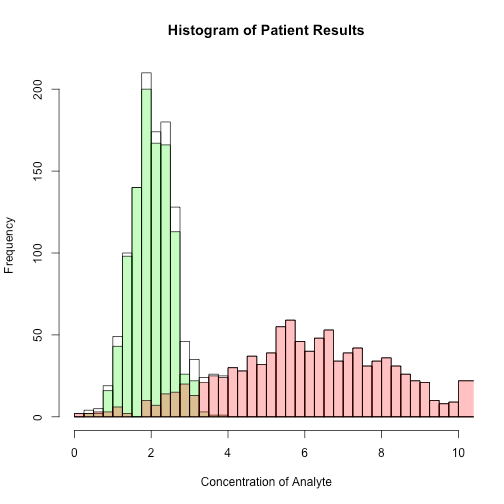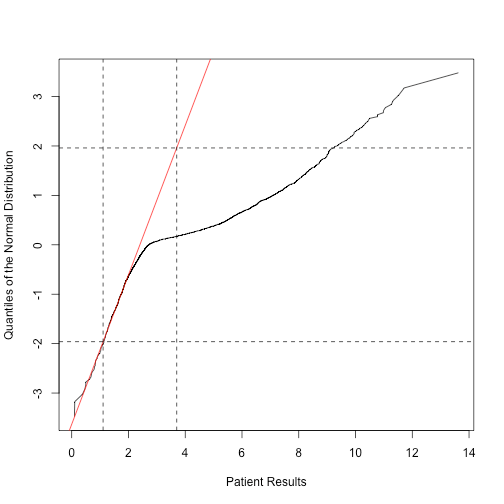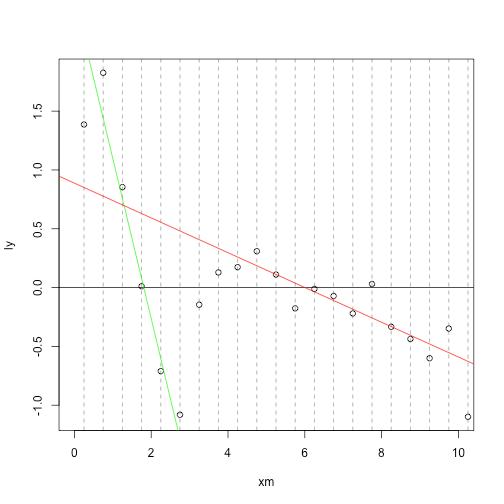Want to share your content on R-bloggers? click here if you have a blog, or here if you don't.

## Background

Let me preface this by saying I am not making a recommendation to use the Hoffman method. Neither am I advocating for reference interval mining from routine data. There are many challenges associated with this kind of effort. That's for another post I think. However, I am going to how one does the calculations for two methods I have seen used: the Hoffman Method and the Bhattacharya Method. Then I will show how to do this using the mixtools package in R which uses the expectation maximum algorithm to determine the maximum likelihood.

## The Concept

When you look at histograms of routine clinical data from allcomers, on some occasions the data will form a bimodal looking distribution formed by the putatively sick and well. If you could statistically determine the distribution of the well subjects, then you could, in principle, determine the reference interval without performing a reference interval study. We can all dream, right?

All three of the approaches I show assume that the two distributions are Gaussian. This is almost never true. But for the purposes of the calculations, I will provide each approach data that meets the assumptions it makes. So, let's make a fake bimodal distribution and see how each method does. We will assume equal numbers of sick and well so that the bimodal distribution is obvious. One will have $$\mu_1 = 2$$ and $$\sigma_1 = 0.5$$ and the other will have $$\mu_2 = 6$$ and $$\sigma_2 = 2$$. The expected normal range for this population is based on $$\mu_1$$ and $$\sigma_1$$ and is $$2 – 0.5 \times 1.96$$ and $$2 + 0.5 \times 1.96$$ or about 1–3.

#two Gaussian distributions with means of 2 and 6 respectively and sd's of 1 and 2
set.seed(10) #to make sure you generate the same data
mode1 <- rnorm(1000,2,0.5)
mode1 <- mode1[mode1 > 0] #get rid of negative results
mode2 <- rnorm(1000,6,2)
mode2 <- mode2[mode2 > 0]
d <- sort(c(mode1,mode2))
dhist <- hist(d,
breaks = c(seq(0,20,0.25),100),
xlim = c(0,10),
main = "Histogram of Patient Results",
xlab = "Concentration of Analyte")To illustrate how the two populations add you can plot one in green and one in pink. The overlap shows in a yucky brown.

hist(d,
breaks = c(seq(0,10,0.25),100),
freq = TRUE,
xlim = c(0,10),
main = "Histogram of Patient Results",
xlab = "Concentration of Analyte")
hist(mode1, breaks = c(seq(0,10,0.25),100), add = TRUE, col = rgb(0,1,0,0.3), freq = TRUE)
hist(mode2, breaks = c(seq(0,10,0.25),100), add = TRUE, col = rgb(1,0,0,0.3), freq = TRUE)## Hoffman

In 1963 Robert Hoffman proposed a simple graphical approach to this problem and use of his method is alive and well—see here for example. The method assumes that both modes are Gaussian and that if one eye-balls (yes…the paper says “eye-fit”) the first linear-looking portion of the cumulative probability distribution (CDF) function as plotted on normal probability paper and finds its intersection with the lines y = 0.05 and y = 0.975, one can impute the normal range.

### What do I plot for Hoffman: a QQ-plot or the CDF?

It is very important to understand that the use normal probability paper, as Hoffman described, was mandatory because it produces a normal probablity plot. As he says,

“This special graph paper serves the useful purpose of 'straightening out' a cumulative gaussian distribution. It forms a straight line.”

A CDF plotted on linear scale is sigmoidal. This is not what we want. We want a normal probability plot which is just a special case of the QQ-plot where the comparator distribution is the normal distribution. Inadvertently plotting a plain old CDF will not produce correct estimates of the lower and upper limits of normal (ie $$\mu \pm 1.96\sigma$$). The reason I emphasize this is that I have seen this error made in a number of reference interval papers—but not the one I cited above—it is correct. The importance of the distinction becomes not-very-subtle when you apply the Hoffman approach to a pure Gaussian distribution. In short, use of the CDF in linear space generates erroneous results as we will show later on.

### The Correct Approach

Here is the standard r-base normal QQ-plot of our mock data set:

qqnorm(d, type = "l")To prevent reader confusion, I am going present the plots the way Hoffman originally showed them. So I will put the patient data on the x-axis. It doesn't change anything. You can do it as you like.

my.qq <- qqnorm(d, datax = TRUE, type = "l", ylab = "Patient Results", xlab = "Quantiles of the Normal Distribution")From this you can see that there is obviously linear section between about x = 0 to x = 2 (and with the eye of faith, there is a second after x = 6). This is what Hoffman calls the “eye-fit”. Since the first linear section is attributable to the first of the two normal distributions which form the overall distribution, we can use the it to determine properties of the first distribution. If I look only at the data between x = 0 and x = 2, I am sort-of guaranteed to be in the first linear section. You don't have to kill yourself to correctly identify where the linearity ends because the density of the points should be highest near the middle of the linear section and this will weight the regression for you.

Next if I extend this line to find its intersection with y = -1.96 and y = 1.96 (ie the z-scores corresponding the limits of normal, namely the 2.5th and 97.5th centiles), I can estimate the reference interval, by dropping perpendicular lines from the two respective intersections. Here is what I get:

#get regression line - it's linear from about from 0 to 2
my.qq <- as.data.frame(my.qq)
linear.bit <- subset(my.qq, x <= 2)
#get the regression line of the linear section
reg <- lm(y ~ x, data = linear.bit)
plot(y ~ x,
data = my.qq,
type = "l",
ylab = "Quantiles of the Normal Distribution",
xlab = "Patient Results")
abline(reg, col = "red")
abline(h = c(-1.96,1.96), lty = 2)
uln.hoff <- unname((1.96 - coef(reg))/coef(reg))
lln.hoff <- unname((-1.96 - coef(reg))/coef(reg))
abline(v = c(lln.hoff,uln.hoff), lty = 2)lln.hoff

##  1.105849

uln.hoff

##  3.699254

So the Hoffman reference interval becomes 1.11 to 3.70 which you can compare to the expected values of about 1 and 3 based on the random data. Not the greatest but not bad.

### What not to do

Let's apply the correct approach to the Hoffman method (QQ-Plot) and incorrect approach (CDF on a linear scale) to a pseudorandom sampling (n=10,000) of the standard normal distribution, which has a mean of 0 and a standard deviation of 1. Therefore the central 95% or “normal range” for this distribution will be -1.96 to 1.96. I will plot regression lines through the linear part of each curve and find the respective intersections with the appropriate horizontal lines.

# QQ-Norm plot of standard normal distribution
z <- sort(rnorm(10000,0,1))
par(mfrow = c(1,2))
my.qq <- qqnorm(z, type = "l", datax = TRUE, plot.it = FALSE)
plot(my.qq,
ylim = c(-2.1,2.1),
type = "l",
main = "Normal QQ-plot",
ylab = "Quantiles of the Normal Distribution",
xlab = "Sample Quantiles")
qqline(z, col = "blue")
abline(h=c(1.96,-1.96), col = "blue")
abline(v = c(1.96,-1.96), col = "blue", lty = 2) #lower and upper limits are -2 and 2.

# CDF of standard normal distribution
my.ecdf <- ecdf(z)
df <- data.frame(z = z, y = my.ecdf(z))
plot(y ~ z, data = df, type = "l", main = "Cumulative Normal Distribution")
abline(v = c(1.96,-1.96), col = "blue", lty = 2)
abline(h = c(0.025,0.975), col = "blue")
linear.bit <- subset(df, z > -.5 & z < 0.5)
abline(lm(y ~ z, data = linear.bit), col = "blue")
abline(v = c(-(0.5 - 0.05/2)*sqrt(2*pi),(0.5 - 0.05/2)*sqrt(2*pi)), col  = rgb(1,0,0,0.5) )The QQ-plot generates estimates the limits of normal, $$\mu \pm 1.96\sigma$$, as about $$\pm 1.96$$ as it should. You can easily show that the same procedure on the CDF intersects the lines $$y = \alpha /2$$ and $$y = 1 – \alpha/2$$ at values of $$\pm (1 – \alpha) \sqrt{\pi/2} \sigma$$ which is about $$\pm 1.19$$ for $$\sigma = 1$$ and $$\alpha = 0.05$$. This erroneous estimate is shown with the pink vertical lines. So the Hoffman method does not work if one attempts to extend the linear portion of the CDF if it is plotted in linear space and it will produce estimates of $$\sigma$$ that are 40% too low. If you're puting this all together, this because the CDF is well away from its linear portion when the cumulative proportions are 0.025 and 0.975—not so for a QQ-plot. If you see a “Hoffman plot” constructed from a sigmoidal CDF plotted on a linear scale, something is wrong.

## Bhattacharya

This method is based on a much more highly cited paper in Biometrics published in 1967 by C.G. Bhattacharya. Loosely speaking, the method of Bhattacharya determines the parameter estimates of $$\mu_i$$ and $$\sigma_i$$ from the slope of the log of the distribution function. It was originally intended as a graphical method and so it also involves some human eye-balling.

We will need the log of the counts from the histogram. When we store the results of a histogram in R, we have the counts automatically.

str(dhist)

## List of 6
##  $breaks : num [1:82] 0 0.25 0.5 0.75 1 1.25 1.5 1.75 2 2.25 ... ##$ counts  : int [1:81] 2 4 5 19 49 100 140 210 174 180 ...
##  $density : num [1:81] 0.004 0.008 0.01 0.038 0.098 ... ##$ mids    : num [1:81] 0.125 0.375 0.625 0.875 1.125 ...
##  $xname : chr "d" ##$ equidist: logi FALSE
##  - attr(*, "class")= chr "histogram"

We can now calculate the log of the counts (denoted $$y$$) and $$\Delta log(y)$$ from bin to bin. We put these in a dataframe along with the counts and the midpoints of the bins. The bin width, which is chosen to be constant $$h$$, is the distance between the midpoints of each bin.

#alter the number of breaks to make the linear sections more obvious.
dhist <- hist(d,
breaks = 30,
plot = FALSE)
ly <- log(dhist$counts) dly <- diff(ly) df <- data.frame(xm = dhist$mids[-length(dhist$mids)], ly = dly, counts = dhist$counts[-length(dhist$mids)]) h <- diff(df$xm)

Now let's plot $$\Delta log(y)$$ as a function of the midpoints of the bins. I also number all the points to facilitate the next step.

plot(ly ~ xm, data = df, xlim = c(0,10))
abline(h = 0)
abline(v = df$xm, lty = 2, col = "#00000080") #number all the points library(calibrate) num.df <- na.omit(df) textxy(num.df$xm, num.df$ly, 1:nrow(num.df), row.names(num.df), cex = 0.8, offset = 1, col = "blue")We can see from the figure that there are two sections where the plot shows a downsloping line: one between points 2 to 6 and another between points 10 to 21. How straight these lines appear is affected by how wide your bins are so if you get lines that are hard to discern, you can try making fewer bins. In any case, using Bhattacharya's notation, the next step in the procedure is do draw regression lines through the $$r_{th}$$ linear section and determine the intercept $$\hat{\lambda}_r$$ with the x-axis. Bhattacharya intended this as a graphical procedure and advises, “While matching the straight line it is better to fit closely to the points where the frequency is large even if the apparent discrepancy becomes somewhat large where the frequency is small.” Since we are doing this by calculation, we can take his advice by weighting the linear regressions according to the counts. This allows the determination of the $$\hat{\mu}_r$$ by: $\hat{\mu}_r = \hat{\lambda}_r + h/2$ and also the determination of $$\hat{\sigma}_r$$ by: $\hat{\sigma}^2_r = -h/ \text{slope}_r – h^2/12$ #linear section 1 linear.bit1 <- subset(df[2:6,]) lm1 <- lm(ly ~ xm, data = linear.bit1, weights = linear.bit1$counts)
lambda1 <- -coef(lm1)/coef(lm1)
mu1 <- lambda1 + h/2
sigma1 <- sqrt(-h/coef(lm1) - h^2/12)

#linear section 2
linear.bit2 <- subset(df[10:21,])
lm2 <- lm(ly ~ xm, data = linear.bit2, weights = linear.bit2$counts) lambda2 <- -coef(lm2)/coef(lm2) mu2 <- lambda2 + h/2 sigma2 <- sqrt(-h/coef(lm2) - h^2/12) #normal range limits lln.bhat <- qnorm(0.025,mu1, sigma1) uln.bhat <- qnorm(0.975,mu1, sigma1) And here are the results we get: mu Values sigma Values Normal Range Limits 2.06 0.59 0.90 6.25 1.83 3.21 And here is what it all looks like plot(ly ~ xm, data = df, xlim = c(0,10)) abline(h = 0) abline(v = df$xm, lty = 2, col = "#00000080")
abline(lm1, col = "green")
abline(lm2, col = "red")In this demonstration, there are only two Gaussian distributions to resolve, but the method is not limited to the resolution of two Gaussian curves at all. If there are more, there will be more downsloping lines crossing the x-axis. So we get normal range estimates of 0.90 and 3.21 which compare much better with the expected values of about 1 and 3. We also get good estimates of $$\mu_2=$$ 6.3 and $$\sigma_2=$$ 1.8 which are about 6 and 2 respectively in our data set.

Bhattacharya also provides a means of calculating the mixing proportion of the two distributions—that is, the proportions of patients in the sick and abnormal populations. We don't need that here so I omit it.

## Gaussian Mixture Model

In R there are a lot of ways to approach the separation of mixtures of distributions using maximum likelihood. Here I am using a function from the mixtools package that is particularly easy to use. The concept of using maximum likelihood for mining your reference interval is not new (see this paper) but many would be intimidated by the math required to do it from scratch.

With R, this is pretty easy but please be cautioned that real data does not play as nice as the data in this demonstration (even moreso for Hoffman and Bhattacharya) and it is unlikely that you will get smashing results unless your data fits the assumptions of the model.

In any case,

#Gaussian Mixed Model - the right way to do this
library(mixtools)
fit <- normalmixEM(d, k = 2) #try to fit two Gaussians

## number of iterations= 28

summary(fit)

## summary of normalmixEM object:
##          comp 1   comp 2
## lambda 0.519121 0.480879
## mu     2.014404 6.186571
## sigma  0.518210 1.966858
## loglik at estimate:  -3961.014

which gives very good parameter estimates indeed! Estimates of $$\mu_1$$ and $$\mu_2$$ are 2.01 and 6.19 respectively and estimates of $$\sigma_1$$ and $$\sigma_2$$ are 0.52 and 1.97 respectively.

Looking at this graphically:

hist(d, freq = FALSE, breaks = 50, main = "Histogram of Patient Results")
#show the respective curves
lines(d,fit$lambda*dnorm(d,fit$mu,fit$sigma), col = "green") lines(d,fit$lambda*dnorm(d,fit$mu,fit$sigma), col = "red")#find the 2.5th 97.5th percentile from the mixed model fit
lln.EM <- qnorm(0.025,fit$mu, fit$sigma)
lln.EM

##  0.9987315

uln.EM <- qnorm(0.975,fit$mu, fit$sigma)
uln.EM

##  3.030077

So the normal range estimate from EM method is 1.00 to 3.03 which is pretty fantastic.

## Summary of Results

LLN ULN
Raw Random Data 1.03 2.98
Hoffman 1.11 3.70
Bhattacharya 0.90 3.21
mixtools EM – winner! 1.00 3.03

It's not too hard to figure out which one of these approaches works best. But what do you do if your patient data distribution is obviously not a mixture of Gaussians (ie when the distributions look skewed)? There are ways to do this in R for this but I will cover that another time–maybe in a paper.

## Conclusion

• Three methods of estimating the normal range from a mixture of Gaussians have been presented.
• The Hoffman method performs OK if you use a QQ-plot.
• The Hoffman method does not work for CDFs plotted on a linear scale.
• The Bhattacharya method performs better but still requires some human oversight.
• The normalmixEM() function from the mixtools package performs very well without any human oversight.
• These results do not imply that any of these approaches will perform well on real patient data for which the components of the overall distribution are not likely to be Gaussian. Caution advised.

Parting Thought

Please don't fall on the wrong side of God's mixture separation procedures for wheat and chaff.

Said, John the Baptist, “But after me comes one who is more powerful than I, whose sandals I am not worthy to carry. He will baptize you with the Holy Spirit and fire. His winnowing fork is in his hand, and he will clear his threshing floor, gathering his wheat into the barn and burning up the chaff with unquenchable fire.”

Matt 3:11–12